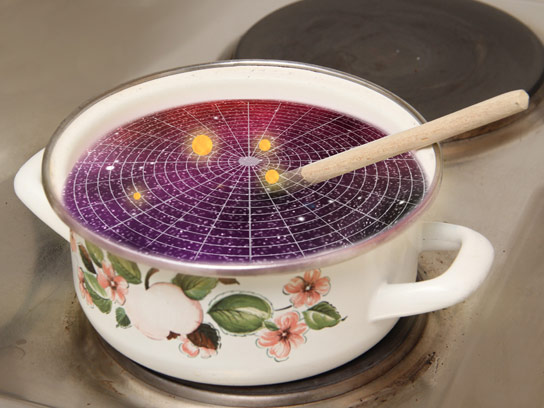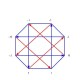# Researchers Reveal How an Expanding Universe Can Emerge Without a “Big Bang”Recipe for a universe: Apply heat and stir. Credit: Vienna University of Technology

In a new study, an international team of researchers mathematically describe how an expanding universe can emerge when time and space are heated, without requiring anything like a “Big Bang.”

When soup is heated, it starts to boil. When time and space are heated, an expanding universe can emerge, without requiring anything like a “Big Bang.” This phase transition between a boring empty space and an expanding universe containing mass has now been mathematically described by a research team at the Vienna University of Technology, together with colleagues from Harvard, the MIT and Edinburgh. The idea behind this result is a remarkable connection between quantum field theory and Einstein’s theory of relativity.

#### A Cookbook for Spacetime

Everybody knows of the transitions between liquid, solid and gaseous phases. But also time and space can undergo a phase transition, as the physicists Steven Hawking and Don Page pointed out in 1983. They calculated that empty space can turn into a black hole at a specific temperature.

Can a similar process create a whole expanding universe such as ours? Daniel Grumiller from the Vienna University of Technology looked into this, together with colleagues from the USA and Great Britain. Their calculations show that there is indeed a critical temperature at which an empty, flat spacetime turns into an expanding universe with mass. “The empty spacetime starts to boil, little bubbles form, one of which expands and eventually takes up all of spacetime,” explains Grumiller.

For this to be possible, the universe has to rotate – so the recipe for creating the universe is “apply heat and stir”. However, the required rotation can be arbitrarily small. In a first step, a spacetime with only two spatial dimensions was considered. “But there is no reason why the same should not be true for a universe with three spatial dimensions,” says Grumiller.

#### Looking for the Structure of the Universe

Our own universe does not seem to have come into existence this way. The phase-transition model is not meant to replace the theory of the Big Bang. “Today, cosmologists know a lot about the early universe – we are not challenging their findings. But we are interested in the question, which phase transitions are possible for time and space and how the mathematical structure of spacetime can be described,” says Grumiller.

The new theory is the logical next step after the so called “AdS-CFT correspondence,” a conjecture put forward in 1997, which has strongly influenced fundamental physics research ever since. It describes a peculiar connection between theories of gravity and quantum field theories – two areas which, at first glance, do not have much in common. In certain limiting cases, according to AdS-CFT correspondence, statements from quantum field theories can be translated into statements concerning gravitational theories and vice versa. This is almost as surprising as the idea of making statements about a stone falling to the ground by actually calculating the temperature of a hot gas. Two completely different areas are being connected – but it works.

In this kind of correspondence, the quantum field theory is always described in one fewer dimension than the gravitational theory. This is called “holographic principle.” Similar to a two dimensional hologram which can depict a three dimensional object, a quantum field theory with two spatial dimensions can describe a physical situation in three spatial dimensions.

#### A Correspondence Principle for Flat Spacetimes

To do this, the gravitational calculations usually have to be done in an exotic kind of geometry – in so-called “Anti-de-Sitter-spaces,” which are quite different from the flat geometry we are used to. However, it has been suspected for a while, that there may be a similar version of the “holographic principle” for flat spacetimes. But for a long time there haven’t been any models showing this.

Last year, Daniel Grumiller and colleagues established such a model (in two spatial dimensions, for simplicity). This led to the current question; phase transitions in quantum field theories are well known. But for symmetry reasons this would mean that gravitational theories should exhibit phase transitions too.

“At first, this was a mystery for us,” says Daniel Grumiller. “This would mean a phase transition between an empty spacetime and an expanding universe. To us, this sounded extremely implausible.” But the calculations showed exactly that. “We are only beginning to understand these remarkable correspondence relations,” says Daniel Grumiller. Which new ideas about our own universe can be derived from this, is hard to say – only spacetime will tell.

References:

PDF Copy of the Studies:

#### 2 Commentson "Researchers Reveal How an Expanding Universe Can Emerge Without a “Big Bang”"

1.Wardell | December 10, 2013 at 8:21 pm | Reply

The Universe we inhabit and measure is not expanding. Hubble’s curvature measures indicated the universe is finite and closed. This is true, the universe is bounded and Invariant in the sense that the change of Gravitationa Energy has a null point 0 =XW = [d/dr,DEL] [-mGM/r, cP]. The Invariant condition is where the velocity v=c.

Physicists need to consider the likelihood that Relativity Theory and Quantum Theory are both wrong. Uniting two wrong Theories will not produce a Unified correct Theory.

I believe the correct Theory is Quaternion Physics,
Total Energy W = -vh/w + cP , where W is the sum of the potential energy and the vector Momentum energy, cP. The vector energy, cP is the so-called “Dark Energy”. The stats of this finite universe are size 155E24 meters, Mass =2E53kg, Power = 3645E49 watts.

The misunderstanding of redshift has misled physicists to erroneously see expansion rather than the refraction coefficient n = c/v. The redshift is the inverse of the refraction. The central attraction force creates the centripetal force and the refraction coefficient. Both the gavity attraction and the atomic nnucleas attraction result in redshift and the same refraction action. The Total Energy is the same W = -vh/w + cP, and the boundary Condition is v/c=1 = 1/n.

The ideas that created the problem are not the ideas to solve the problem.

•jack | December 15, 2013 at 6:02 pm | Reply

I did not understand anything you just said haha
But I want to know more, the redshift I thought was when a galaxy was moving, coming towards us was blue and red was moving away.
Now explain why quantum physics is the right theory without all the intense mathematics.